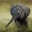Related Tags

c++
sorting

# How to arrange non-negative integers to form the largest numberChayshta Singh

## Overview

This Answer will teach you how to arrange a list of non-negative integers (including zero) in a way that they form the largest number possible. We'll output the resultant number as a string.

The algorithm should run in $O(N log N)$ time where $N$ is the size of the list.

### Algorithm

If x and y are two integers, let x_y represent an integer formed by appending y to x. Similarly, y_x means appending x to y.

Our sorting logic depends on the comparison of these combined integers. For any integers a and b in the list, if a_b is greater than b_a, we want to position a ahead of b and vice-versa. After this is true for all pairs of integers, we'll get the arrangement that forms the largest number.

Consider a list of two elements {91, 9}. The two combined numbers that can be formed are 919and 991. The correct order of the list is {9, 91} because appending all integers of the list in this order creates the largest number,991.

This algorithm can be implemented by sorting the list by using the sort function in the algorithm library with a custom comparator. This will take $O(N log N)$ time to sort a list of N integers.

//calculates 10 raised to the power of n
unsigned long long pow10Int(int n);

//returns the number of digits in num in base 10
int numDigits(int const num);

//custom comparator
//returns true when a should be placed left to b i.e a_b > b_a and vice-versa
bool combinedNumComp(int const & a, int const & b){
int digitsB = numDigits(b);     //finds number of digits in b
auto a_b = a * pow10Int(digitsB) + b;   //add zeroes at the end of a and then add b

int digitsA = numDigits(a);     //finds number of digits in a
auto b_a = b * pow10Int(digitsA) + a;   //add zeroes at the end of b and then add a

return a_b > b_a;
}
Custom comparator

The comparator is called with the sort function.

vector<int> lists{9,8,81,89};
sort(lists.begin(), lists.end(), combinedNumComp);

### Implementation details

1. The number of digits of a number $x$ in base $b$ is given by $[log_b(x)] +1$.
2. We use unsigned long long to hold the combined integers. An unsigned long long type of size 8 bytes can store a maximum value of approximately 1.8 * 1019. This limits our input integer to have a maximum value of 1.8 * 109.

### Code example

#include <iostream>
#include <cmath>
#include <vector>
#include <algorithm>
#include <string>
using namespace std;

//calculates 10 raised to the power of n
unsigned long long pow10Int(int n){
if(n == 0) return 1ull; //ul : numeric literal for unsigned long long
unsigned long long rs = 1ull;
while(n){
rs *= 10;
n--;
}
return rs;
}

//returns the number of digits in num in base 10
int numDigits(int const num){
return static_cast<int>(log10(num) + 1);
}

//custom comparator
//returns true when a should be placed left to b i.e a_b > b_a and vice-versa
bool combinedNumComp(int const & a, int const & b){
int digitsB = numDigits(b);     //finds number of digits in b
auto a_b = a * pow10Int(digitsB) + b;   //add zeroes at the end of a and then add b

int digitsA = numDigits(a);     //finds number of digits in a
auto b_a = b * pow10Int(digitsA) + a;   //add zeroes at the end of b and then add a

return a_b > b_a;
}

//returns a string formed by appending all ints in v
string createString(vector<int> & v){
string s = "";
for(auto ele : v){
s += to_string(ele);    //converts ele from int to string and append it to s
}
return s;
}

int main(){
vector<int> lists{9, 91, 89, 9,8,99,78};
cout <<"Input : ";
for_each(lists.begin(), lists.end(), [](int a){std::cout << a << ", " ;}); //print using lambda function
sort(lists.begin(), lists.end(), combinedNumComp);  //sort using comparator numComp
cout <<"\nRearranged List : ";
for_each(lists.begin(), lists.end(), [](int a){std::cout << a << ", " ;}); //print using lambda function
cout <<"\nOutput String : ";
cout << createString(lists);    //output the string
return 0;
}
C++ code: Find largest number by arranging non-negative integers in a list

### Time complexity

The time complexity of the algorithm is $O(N log N)$ which is the time taken to sort a list of $N$ elements.

### Explanation

Line 9–17: Implements a function to calculate 10 raised to the power of n. This is used when forming the combined string a_b.

Line 20: A trivial function to get the number of digits in num in base 10. It is implemented separately for better readability.

Line 26–33: Defines a comparator function used within sort.

Line 39: auto is deduced here as int.

Line 48: Uses a lambda function within for_each to print the vector.

Line 49: Sorts the list with sort() and combinedNumComp() .

Line 53: Creates the resultant string and outputs it.

RELATED TAGS

c++
sorting

CONTRIBUTORChayshta Singh
RELATED COURSES

View all Courses

Keep Exploring

Learn in-demand tech skills in half the time